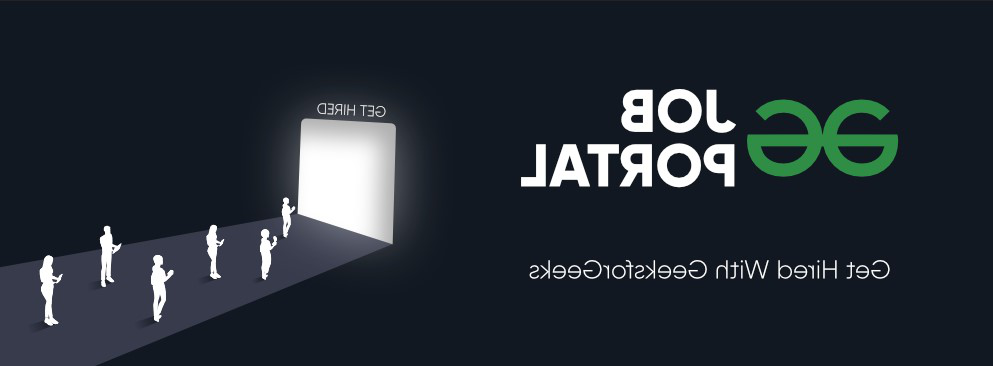GFG App
Open AppBrowser
Continue

# Python PyTorch – RandomHorizontalFlip() Function

In this article, we will discuss the RandomHorizontalFlip() Method in PyTorch Python.

## RandomHorizontalFlip() method

RandomHorizontalFlip() method of torchvision.transforms module is used to horizontally flip the given image at a random angle with a given probability. This method accepts a PIL and tensor image as input. The tensor image is a PyTorch tensor with shape [C, H, W], where C represents the number of channels and  H, W represents the height and width respectively. This method returns a horizontally flipped image and an original image if the probability P is 1 or 0 respectively, if P is in the range between 0 to 1 then P is the probability to return the horizontally flipped image.

Syntax: torchvision.transforms.RandomHorizontalFlip(p)(img)

Parameter:

• p: p is the probability of the image being flipped at a random angle.
• img: input image to be flipped.

Returns: This method returns a randomly flipped image  at a random angle.

The below image is used for demonstration:### Example 1:

In this example, we flip an image using RandomHorizontalFlip() Method when the probability is 1.

## Python3

 `# import required libraries ` `import` `torch ` `import` `torchvision.transforms as T ` `from` `PIL ``import` `Image ` ` `  `# read input image from computer ` `img ``=` `Image.``open``(``'a.png'``) ` ` `  `# define a transform ` `transform ``=` `T.RandomHorizontalFlip(p``=``1``) ` ` `  `# apply above defined transform to  ` `# input image ` `img ``=` `transform(img) ` ` `  `# display result ` `img.show() `

Output:### Example 2:

In this example, we flip an image using RandomHorizontalFlip() Method when the probability is in the range of 0 to 1.

## Python3

 `# import required libraries ` `import` `torch ` `import` `torchvision.transforms as T ` `from` `PIL ``import` `Image ` ` `  `# read input image from computer ` `img ``=` `Image.``open``(``'img.png'``) ` ` `  `# define a transform ` `transform ``=` `T.RandomHorizontalFlip(p``=``0.5``) ` ` `  `# apply above defined transform to  ` `# input image ` `img ``=` `transform(img) ` ` `  `# display result ` `img.show() `

Output:My Personal Notes arrow_drop_up